# Specifications

## Specifications

Europe

### Three-phase 200V series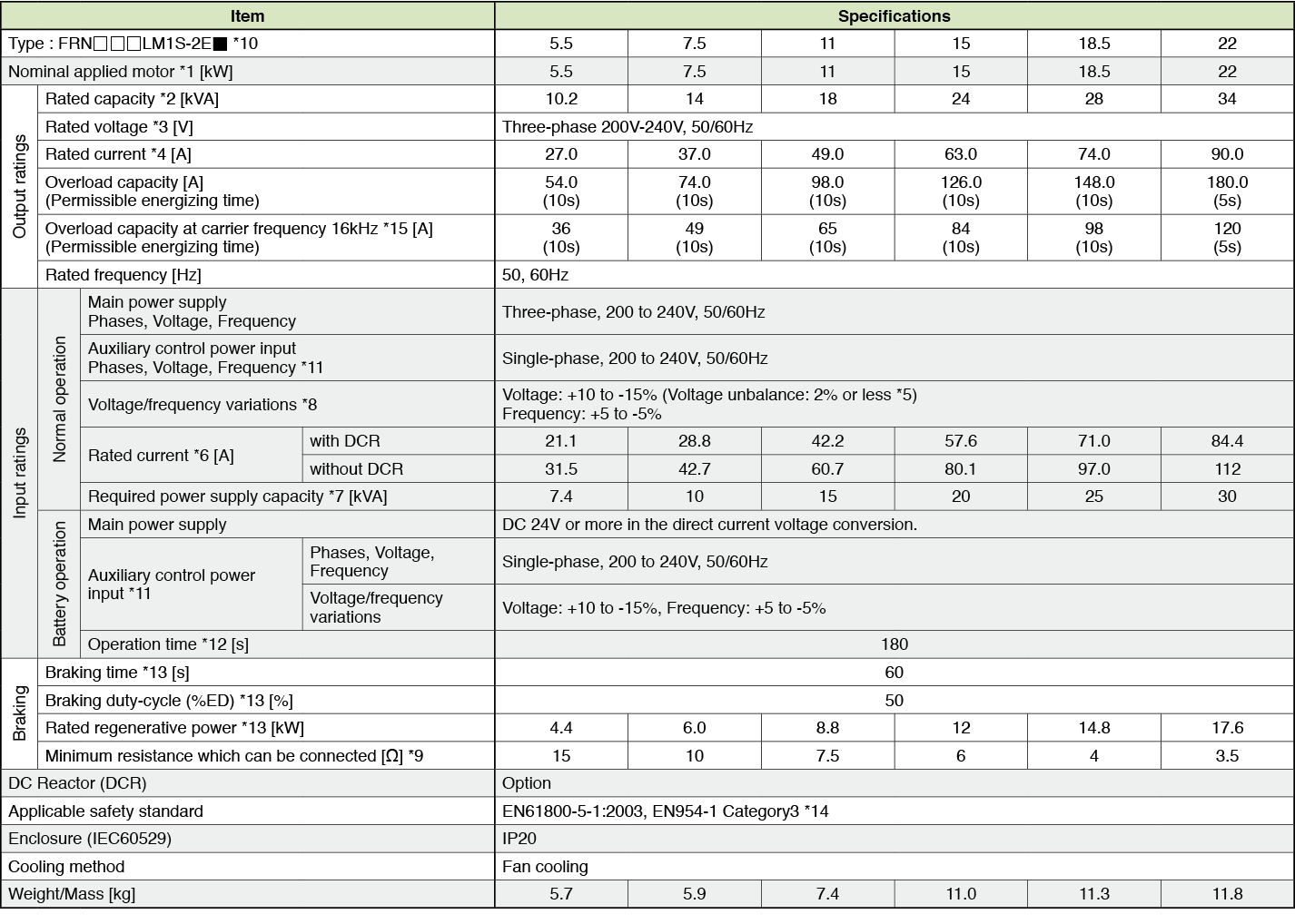*1)
Fuji’s 4-pole standard motor
*2)
Rated capacity is calculated by regarding the output rated voltage as 220V for three-phase 200V series.
*3)
Output voltage cannot exceed the power supply voltage.
*4)
It is a value in the condition of the career frequency 10kHz and the ambient temperature 45°C. Select the inverter capacity such that the square average current in cycle operation is 80% or less of the rated current of an inverter.
*5)
Voltage unbalance [%] = (Max. voltage [V] - Min. voltage [V])/ Three-phase average voltage [V] x 67 (IEC61800-3)
*6)
The power supply capacity is 500kVA (ten times the inverter capacity when the inverter capacity exceeds 50kVA), and the calculation value when connecting with the power supply of %X=5%.
*7)
Obtained when a DC Reactor is used.
*8)
An acceptable variation of the main power supply and the control power supply assistance input.
*9)
The admissible error of minimum resistance is ±5%.
*10)
 Type of inverter Description FRN□□□LM1S-2E CAN version FRN□□□LM1S-2EA DCP version
*11)
The same AC power as the main power supply input is connected for the backup of the control circuit power source.
*12)
It is a value in the condition of the career frequency 10kHz and the ambient temperature 45°C. Use the inverter such that the square average current in battery operation is 80% or less of the rated current of an inverter.
*13)
Braking time and duty cycle (%ED) are defined by cycle operation at the rated regenerative power as shown in the figure below.
*14)
The inverter that last 2 digits of a software version are from 50 to 99 corresponds to this standard.
*15)
When output exceeds this overload capacity at carrier frequency 16kHz, carrier frequency is reduced automatically. The reduced carrier frequency is maintained until an inverter stops.

### Three-phase 400V series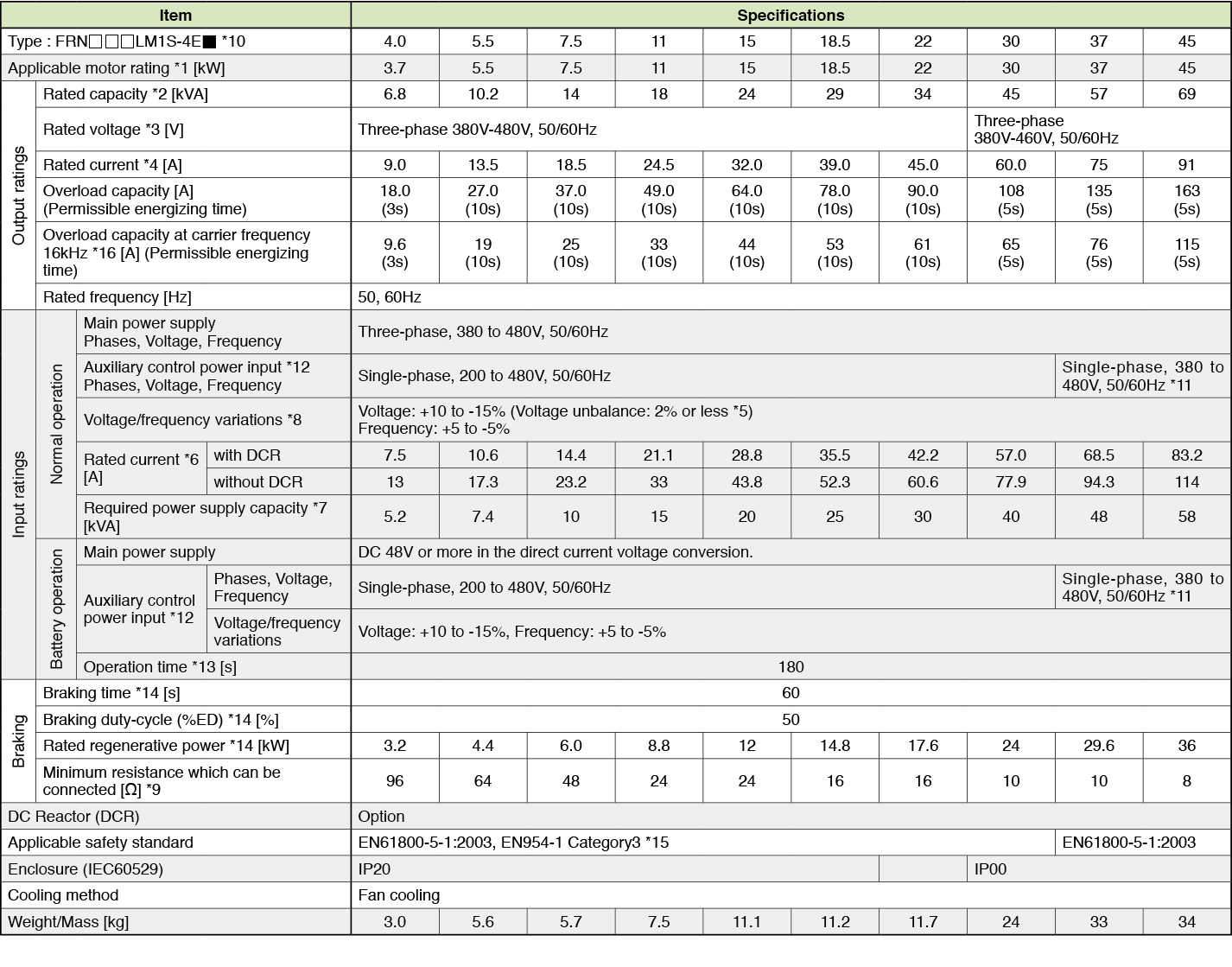*1)
Fuji’s 4-pole standard motor
*2)
Rated capacity is calculated by regarding the output rated voltage as 440V for three-phase 400V series.
*3)
Output voltage cannot exceed the power supply voltage.
*4)
It is a value in the condition of the career frequency 10kHz and the ambient temperature 45°C. Select the inverter capacity such that the square average current in cycle operation is 80% or less of the rated current of an inverter.
*5)
Voltage unbalance [%] = (Max. voltage [V] - Min. voltage [V])/ Three-phase average voltage [V] x 67 (IEC61800-3)
*6)
The power supply capacity is 500kVA (ten times the inverter capacity when the inverter capacity exceeds 50kVA), and the calculation value when connecting with the power supply of %X=5%.
*7)
Obtained when a DC Reactor is used.
*8)
An acceptable variation of the main power supply and the control power supply assistance input.
*9)
The admissible error of minimum resistance is ±5%.
*10)
 Type of inverter Description FRN□□□LM1S-4E CAN version FRN□□□LM1S-4EA DCP version
*11)
It is necessary to change the power-supply voltage change connector on the power supply printed wiring board depend on the power-supply voltage.
*12)
30kW or less
The same AC power as the main power supply input is connected for the backup of the control circuit power source.
37kW or more
The same AC power as the main power supply input is connected for the control circuit, the fan, and the contactor.
The inverter doesn’t operate if the power supply is not input to the auxiliary control power input. Please supply power.
*13)
It is a value in the condition of the career frequency 10kHz and the ambient temperature 45°C. Use the inverter such that the square average current in battery operation is 80% or less of the rated current of an inverter.
*14)
Braking time and duty cycle (%ED) are defined by cycle operation at the rated regenerative power as shown in the figure below.
*15)
The inverter that last 2 digits of a software version are from 50 to 99 corresponds to this standard.
*16)
When output exceeds this overload capacity at carrier frequency 16kHz, carrier frequency is reduced automatically. The reduced carrier frequency is maintained until an inverter stops.

### Single-phase 200V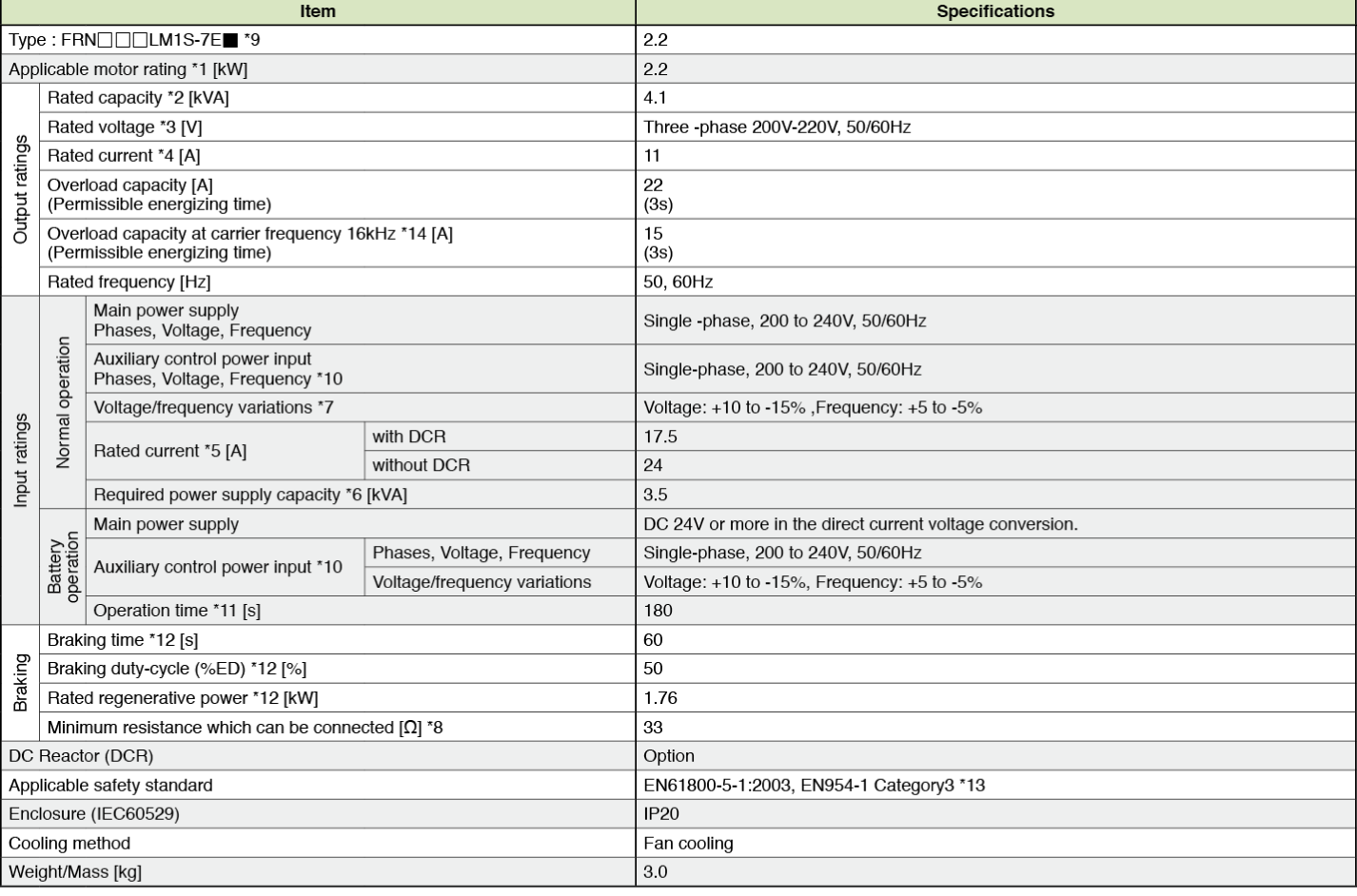*1)
Fuji’s 4-pole standard motor
*2)
Rated capacity is calculated by regarding the output rated voltage as 220V.
*3)
Output voltage cannot exceed the power supply voltage.
*4)
It is a value in the condition of the career frequency 10kHz and the ambient temperature 45°C. Select the inverter capacity such that the square average current in cycle operation is 80% or less of the rated current of an inverter.
*5)
The power supply capacity is 500kVA (ten times the inverter capacity when the inverter capacity exceeds 50kVA), and the calculation value when connecting with the power supply of %X=5%.
*6)
Obtained when a DC Reactor is used.
*7)
An acceptable variation of the main power supply and the control power supply assistance input.
*8)
The admissible error of minimum resistance is ±5%.
*9)
 Type of inverter Description FRN□□□LM1S-7E CAN version FRN□□□LM1S-7EA DCP version
*10)
The same AC power as the main power supply input is connected for the backup of the control circuit power source.
*11)
It is a value in the condition of the career frequency 10kHz and the ambient temperature 45°C. Use the inverter such that the square average current in battery operation is 80% or less of the rated current of an inverter.
*12)
Braking time and duty cycle (%ED) are defined by cycle operation at the rated regenerative power as shown in the figure below.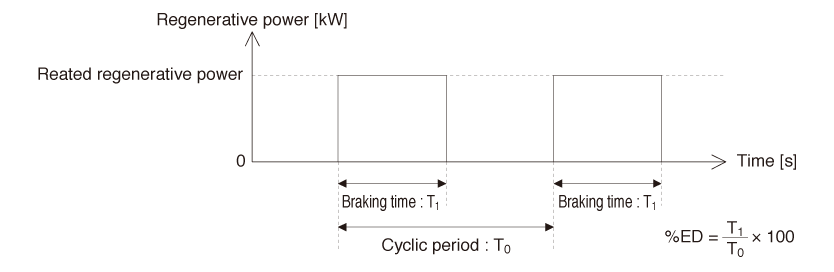*13)
The inverter that last 2 digits of a software version are from 50 to 99 corresponds to this standard.
*14)
When output exceeds this overload capacity at carrier frequency 16kHz, carrier frequency is reduced automatically. The reduced carrier frequency is maintained until an inverter stops.
China

### Three-phase 400V series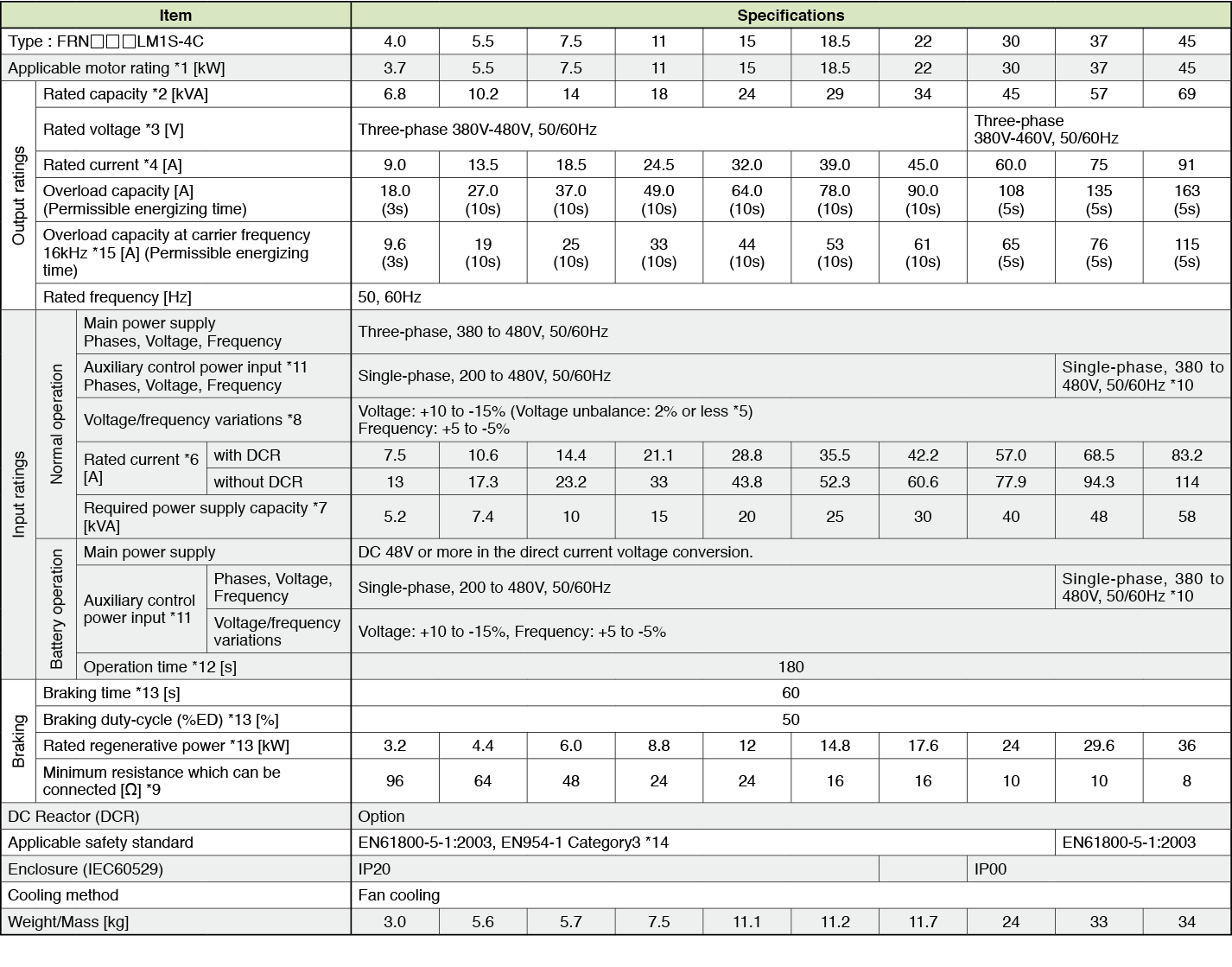*1)
Fuji’s 4-pole standard motor
*2)
Rated capacity is calculated by regarding the output rated voltage as 440V for three-phase 400V series.
*3)
Output voltage cannot exceed the power supply voltage.
*4)
It is a value in the condition of the career frequency 10kHz and the ambient temperature 45°C. Select the inverter capacity such that the square average current in cycle operation is 80% or less of the rated current of an inverter.
*5)
Voltage unbalance [%] = (Max. voltage [V] - Min. voltage [V])/ Three-phase average voltage [V] x 67 (IEC61800-3)
*6)
The power supply capacity is 500kVA (ten times the inverter capacity when the inverter capacity exceeds 50kVA), and the calculation value when connecting with the power supply of %X=5%.
*7)
Obtained when a DC Reactor is used.
*8)
An acceptable variation of the main power supply and the control power supply assistance input.
*9)
The admissible error of minimum resistance is ±5%.
*10)
It is necessary to change the power-supply voltage change connector on the power supply printed wiring board depend on the power-supply voltage.
*11)
30kW or less
The same AC power as the main power supply input is connected for the backup of the control circuit power source.
37kW or more
The same AC power as the main power supply input is connected for the control circuit, the fan, and the contactor.
The inverter doesn’t operate if the power supply is not input to the auxiliary control power input. Please supply power.
*12)
It is a value in the condition of the career frequency 10kHz and the ambient temperature 45°C. Use the inverter such that the square average current in battery operation is 80% or less of the rated current of an inverter.
*13)
Braking time and duty cycle (%ED) are defined by cycle operation at the rated regenerative power as shown in the figure below.
*14)
The inverter that last 2 digits of a software version are from 50 to 99 corresponds to this standard.
*15)
When output exceeds this overload capacity at carrier frequency 16kHz, carrier frequency is reduced automatically. The reduced carrier frequency is maintained until an inverter stops.
Asia

### Three-phase 200V series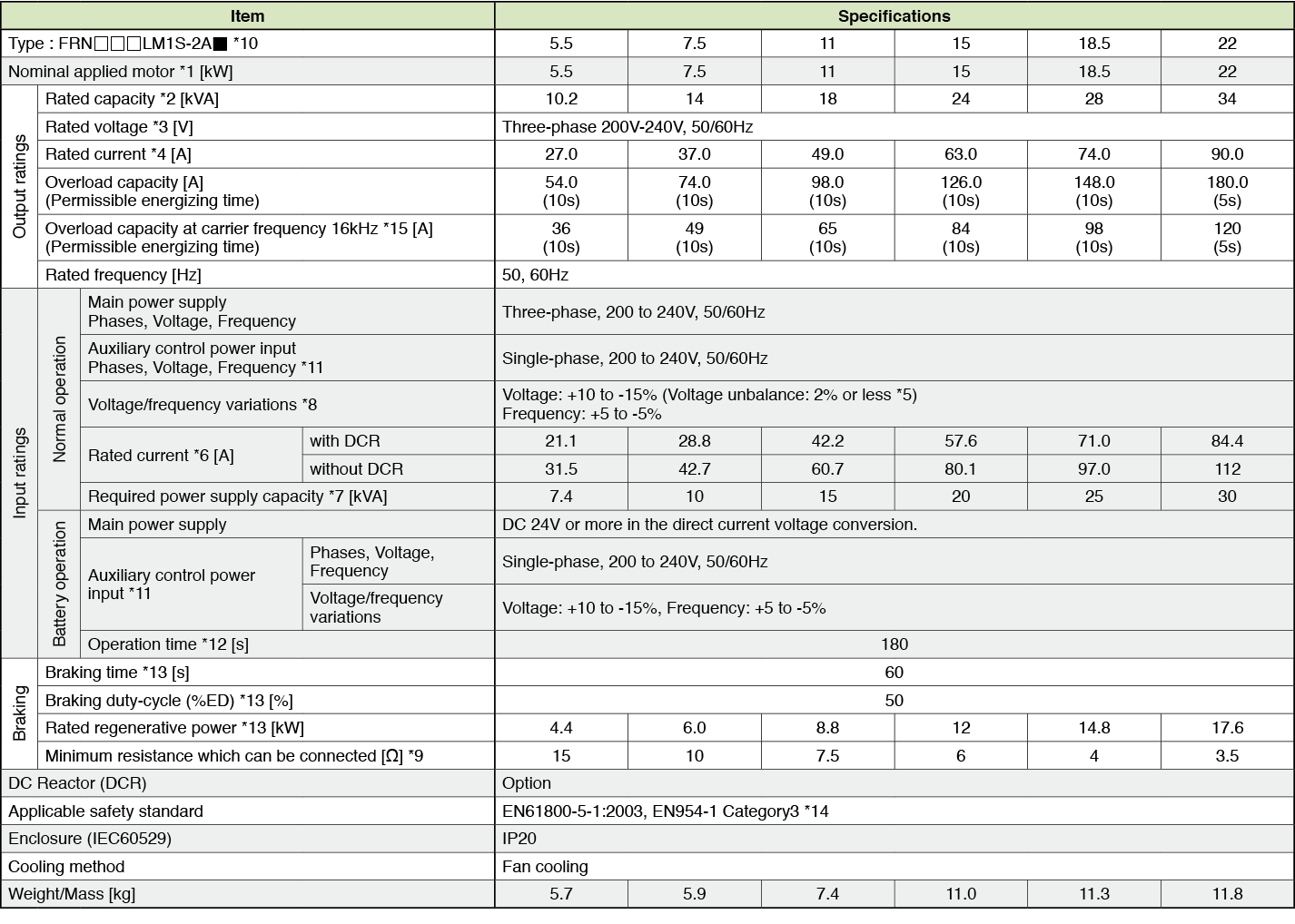*1)
Fuji’s 4-pole standard motor
*2)
Rated capacity is calculated by regarding the output rated voltage as 220V for three-phase 200V series.
*3)
Output voltage cannot exceed the power supply voltage.
*4)
It is a value in the condition of the career frequency 10kHz and the ambient temperature 45°C. Select the inverter capacity such that the square average current in cycle operation is 80% or less of the rated current of an inverter.
*5)
Voltage unbalance [%] = (Max. voltage [V] - Min. voltage [V])/ Three-phase average voltage [V] x 67 (IEC61800-3)
*6)
The power supply capacity is 500kVA (ten times the inverter capacity when the inverter capacity exceeds 50kVA), and the calculation value when connecting with the power supply of %X=5%.
*7)
Obtained when a DC Reactor is used.
*8)
An acceptable variation of the main power supply and the control power supply assistance input.
*9)
The admissible error of minimum resistance is ±5%.
*10)
 Type of inverter Description FRN□□□LM1S-2A CAN version FRN□□□LM1S-2AA DCP version
*11)
The same AC power as the main power supply input is connected for the backup of the control circuit power source.
*12)
It is a value in the condition of the career frequency 10kHz and the ambient temperature 45°C. Use the inverter such that the square average current in battery operation is 80% or less of the rated current of an inverter.
*13)
Braking time and duty cycle (%ED) are defined by cycle operation at the rated regenerative power as shown in the figure below.
*14)
The inverter that last 2 digits of a software version are from 50 to 99 corresponds to this standard.
*15)
When output exceeds this overload capacity at carrier frequency 16kHz, carrier frequency is reduced automatically. The reduced carrier frequency is maintained until an inverter stops.

### Three-phase 400V series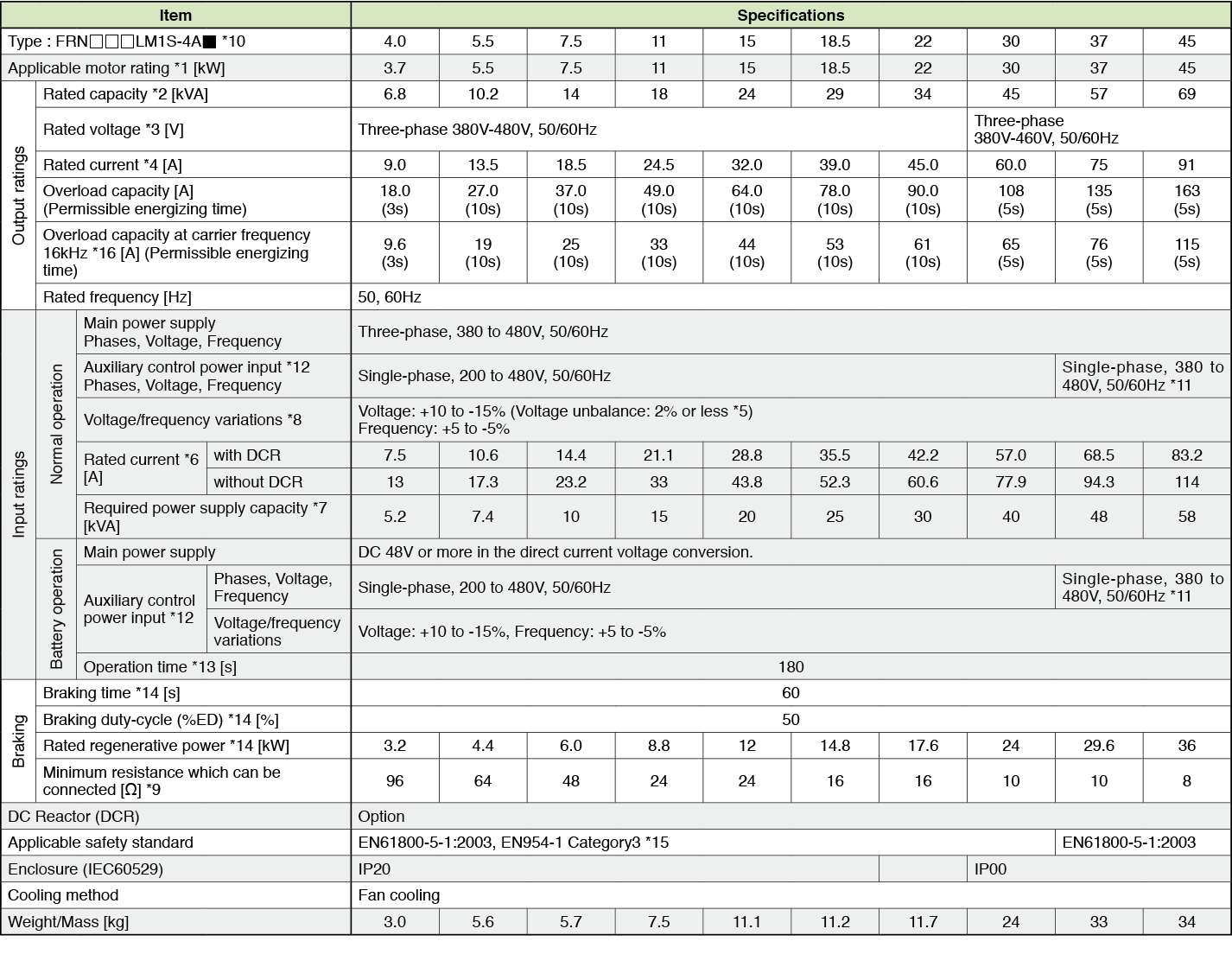*1)
Fuji’s 4-pole standard motor
*2)
Rated capacity is calculated by regarding the output rated voltage as 440V for three-phase 400V series.
*3)
Output voltage cannot exceed the power supply voltage.
*4)
It is a value in the condition of the career frequency 10kHz and the ambient temperature 45°C. Select the inverter capacity such that the square average current in cycle operation is 80% or less of the rated current of an inverter.
*5)
Voltage unbalance [%] = (Max. voltage [V] - Min. voltage [V])/ Three-phase average voltage [V] x 67 (IEC61800-3)
*6)
The power supply capacity is 500kVA (ten times the inverter capacity when the inverter capacity exceeds 50kVA), and the calculation value when connecting with the power supply of %X=5%.
*7)
Obtained when a DC Reactor is used.
*8)
An acceptable variation of the main power supply and the control power supply assistance input.
*9)
The admissible error of minimum resistance is ±5%.
*10)
 Type of inverter Description FRN□□□LM1S-4A CAN version FRN□□□LM1S-4AA DCP version
*11)
It is necessary to change the power-supply voltage change connector on the power supply printed wiring board depend on the power-supply voltage.
*12)
30kW or less
The same AC power as the main power supply input is connected for the backup of the control circuit power source.
37kW or more
The same AC power as the main power supply input is connected for the control circuit, the fan, and the contactor.
The inverter doesn’t operate if the power supply is not input to the auxiliary control power input. Please supply power.
*13)
It is a value in the condition of the career frequency 10kHz and the ambient temperature 45°C. Use the inverter such that the square average current in battery operation is 80% or less of the rated current of an inverter.
*14)
Braking time and duty cycle (%ED) are defined by cycle operation at the rated regenerative power as shown in the figure below.
*15)
The inverter that last 2 digits of a software version are from 50 to 99 corresponds to this standard.
*16)
When output exceeds this overload capacity at carrier frequency 16kHz, carrier frequency is reduced automatically. The reduced carrier frequency is maintained until an inverter stops.

### Single-phase 200V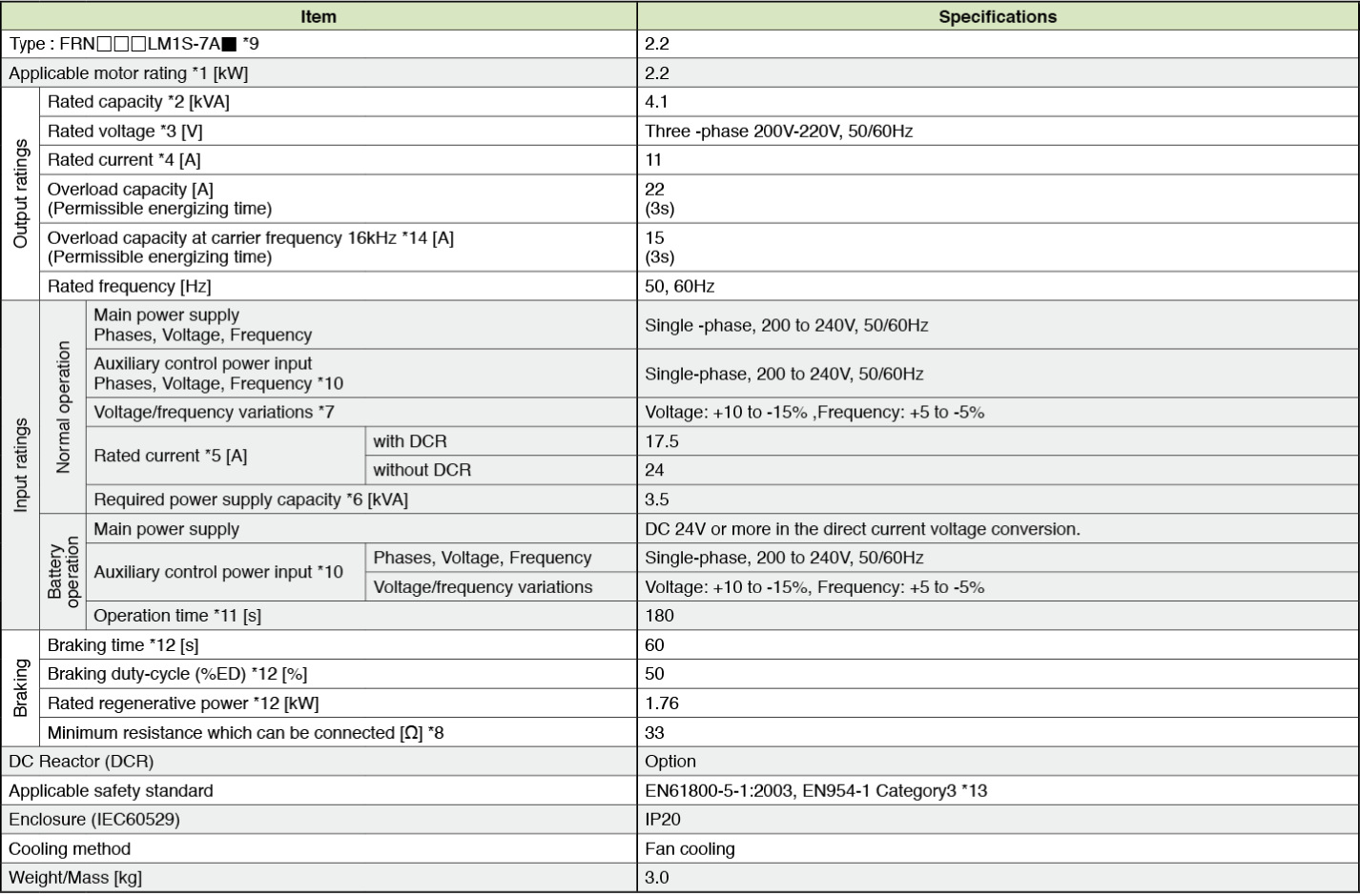*1)
Fuji’s 4-pole standard motor
*2)
Rated capacity is calculated by regarding the output rated voltage as 220V.
*3)
Output voltage cannot exceed the power supply voltage.
*4)
It is a value in the condition of the career frequency 10kHz and the ambient temperature 45°C. Select the inverter capacity such that the square average current in cycle operation is 80% or less of the rated current of an inverter.
*5)
The power supply capacity is 500kVA (ten times the inverter capacity when the inverter capacity exceeds 50kVA), and the calculation value when connecting with the power supply of %X=5%.
*6)
Obtained when a DC Reactor is used.
*7)
An acceptable variation of the main power supply and the control power supply assistance input.
*8)
The admissible error of minimum resistance is ±5%.
*9)
 Type of inverter Description FRN□□□LM1S-7A CAN version FRN□□□LM1S-7AA DCP version
*10)
The same AC power as the main power supply input is connected for the backup of the control circuit power source.
*11)
It is a value in the condition of the career frequency 10kHz and the ambient temperature 45°C. Use the inverter such that the square average current in battery operation is 80% or less of the rated current of an inverter.
*12)
Braking time and duty cycle (%ED) are defined by cycle operation at the rated regenerative power as shown in the figure below.*13)
The inverter that last 2 digits of a software version are from 50 to 99 corresponds to this standard.
*14)
When output exceeds this overload capacity at carrier frequency 16kHz, carrier frequency is reduced automatically. The reduced carrier frequency is maintained until an inverter stops.
Taiwan

### Three-phase 200V series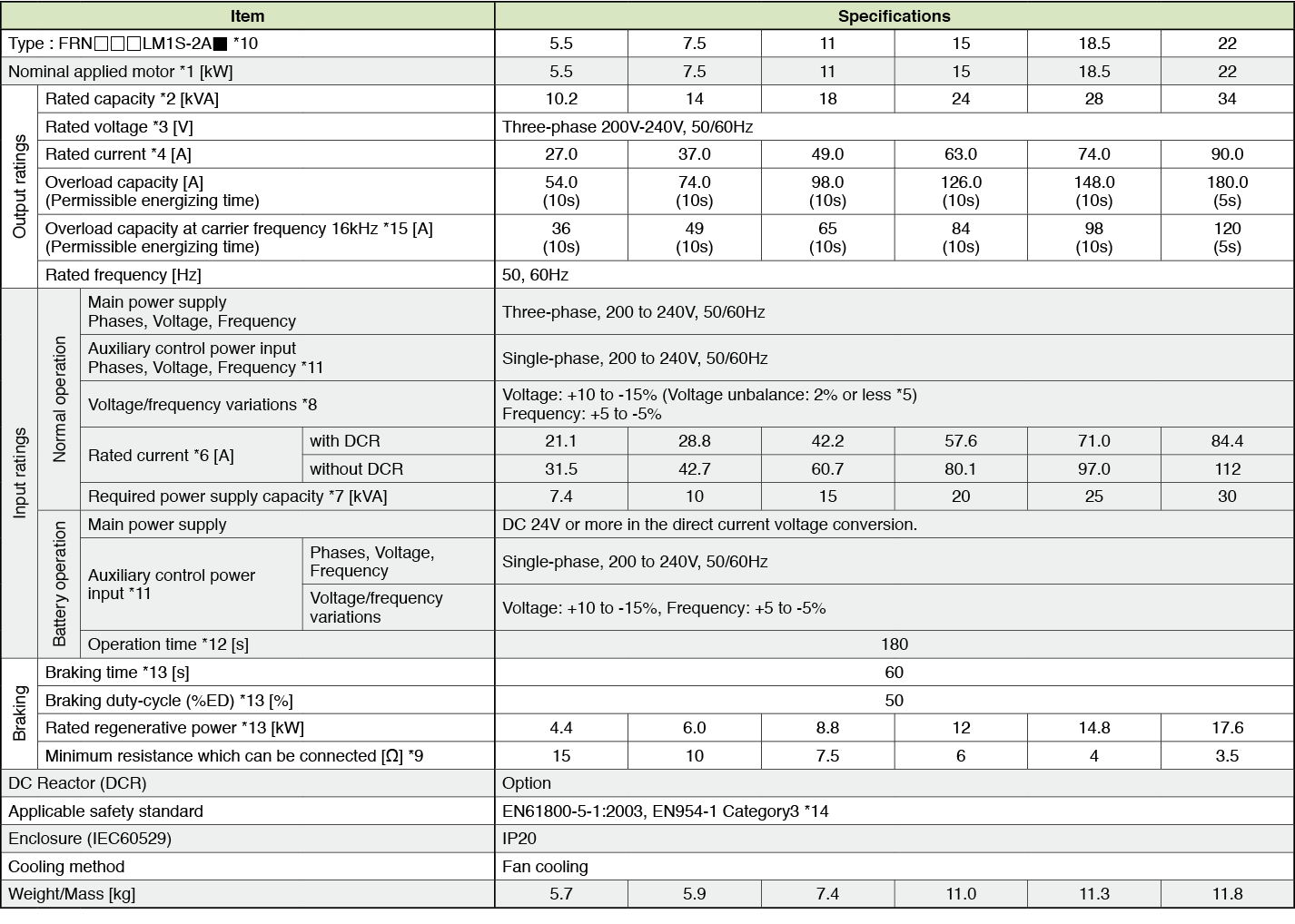*1)
Fuji’s 4-pole standard motor
*2)
Rated capacity is calculated by regarding the output rated voltage as 220V for three-phase 200V series.
*3)
Output voltage cannot exceed the power supply voltage.
*4)
It is a value in the condition of the career frequency 10kHz and the ambient temperature 45°C. Select the inverter capacity such that the square average current in cycle operation is 80% or less of the rated current of an inverter.
*5)
Voltage unbalance [%] = (Max. voltage [V] - Min. voltage [V])/ Three-phase average voltage [V] x 67 (IEC61800-3)
*6)
The power supply capacity is 500kVA (ten times the inverter capacity when the inverter capacity exceeds 50kVA), and the calculation value when connecting with the power supply of %X=5%.
*7)
Obtained when a DC Reactor is used.
*8)
An acceptable variation of the main power supply and the control power supply assistance input.
*9)
The admissible error of minimum resistance is ±5%.
*10)
 Type of inverter Description FRN□□□LM1S-2A CAN version FRN□□□LM1S-2AA DCP version
*11)
The same AC power as the main power supply input is connected for the backup of the control circuit power source.
*12)
It is a value in the condition of the career frequency 10kHz and the ambient temperature 45°C. Use the inverter such that the square average current in battery operation is 80% or less of the rated current of an inverter.
*13)
Braking time and duty cycle (%ED) are defined by cycle operation at the rated regenerative power as shown in the figure below.
*14)
The inverter that last 2 digits of a software version are from 50 to 99 corresponds to this standard.
*15)
When output exceeds this overload capacity at carrier frequency 16kHz, carrier frequency is reduced automatically. The reduced carrier frequency is maintained until an inverter stops.

### Three-phase 400V series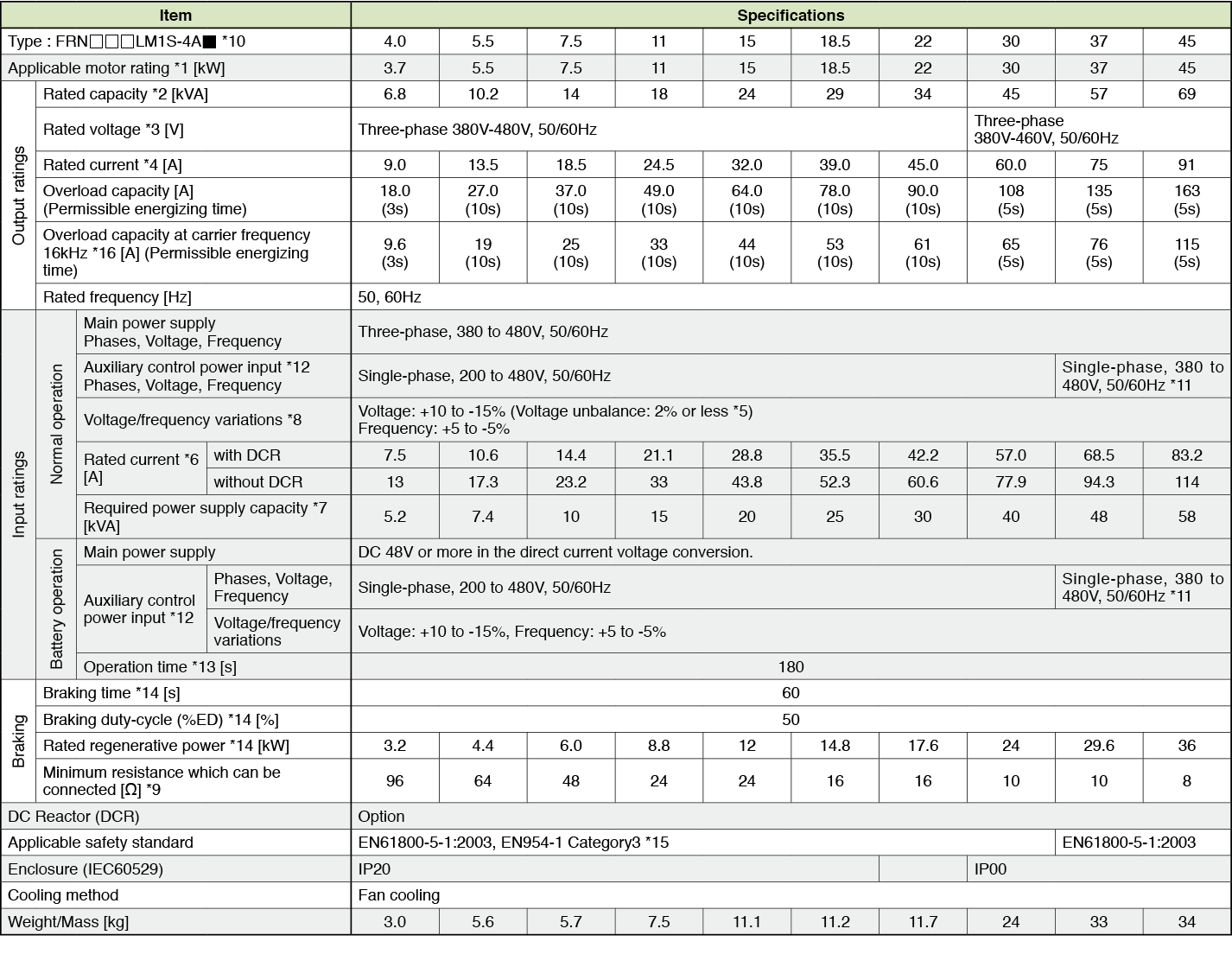*1)
Fuji’s 4-pole standard motor
*2)
Rated capacity is calculated by regarding the output rated voltage as 440V for three-phase 400V series.
*3)
Output voltage cannot exceed the power supply voltage.
*4)
It is a value in the condition of the career frequency 10kHz and the ambient temperature 45°C. Select the inverter capacity such that the square average current in cycle operation is 80% or less of the rated current of an inverter.
*5)
Voltage unbalance [%] = (Max. voltage [V] - Min. voltage [V])/ Three-phase average voltage [V] x 67 (IEC61800-3)
*6)
The power supply capacity is 500kVA (ten times the inverter capacity when the inverter capacity exceeds 50kVA), and the calculation value when connecting with the power supply of %X=5%.
*7)
Obtained when a DC Reactor is used.
*8)
An acceptable variation of the main power supply and the control power supply assistance input.
*9)
The admissible error of minimum resistance is ±5%.
*10)
 Type of inverter Description FRN□□□LM1S-4A CAN version FRN□□□LM1S-4AA DCP version
*11)
It is necessary to change the power-supply voltage change connector on the power supply printed wiring board depend on the power-supply voltage.
*12)
30kW or less
The same AC power as the main power supply input is connected for the backup of the control circuit power source.
37kW or more
The same AC power as the main power supply input is connected for the control circuit, the fan, and the contactor.
The inverter doesn’t operate if the power supply is not input to the auxiliary control power input. Please supply power.
*13)
It is a value in the condition of the career frequency 10kHz and the ambient temperature 45°C. Use the inverter such that the square average current in battery operation is 80% or less of the rated current of an inverter.
*14)
Braking time and duty cycle (%ED) are defined by cycle operation at the rated regenerative power as shown in the figure below.
*15)
The inverter that last 2 digits of a software version are from 50 to 99 corresponds to this standard.
*16)
When output exceeds this overload capacity at carrier frequency 16kHz, carrier frequency is reduced automatically. The reduced carrier frequency is maintained until an inverter stops.

### Single-phase 200V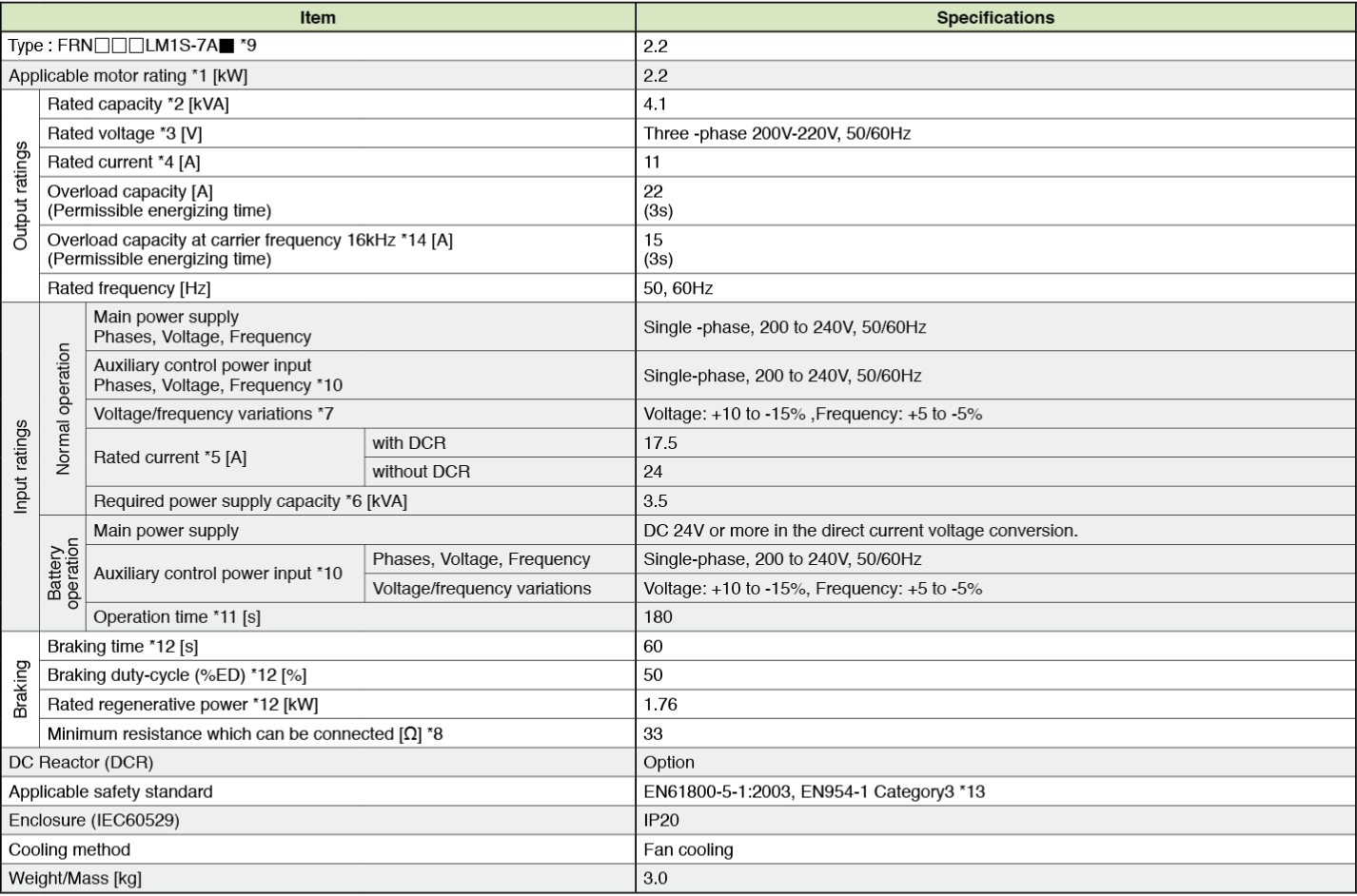*1)
Fuji’s 4-pole standard motor
*2)
Rated capacity is calculated by regarding the output rated voltage as 220V.
*3)
Output voltage cannot exceed the power supply voltage.
*4)
It is a value in the condition of the career frequency 10kHz and the ambient temperature 45°C. Select the inverter capacity such that the square average current in cycle operation is 80% or less of the rated current of an inverter.
*5)
The power supply capacity is 500kVA (ten times the inverter capacity when the inverter capacity exceeds 50kVA), and the calculation value when connecting with the power supply of %X=5%.
*6)
Obtained when a DC Reactor is used.
*7)
An acceptable variation of the main power supply and the control power supply assistance input.
*8)
The admissible error of minimum resistance is ±5%.
*9)
 Type of inverter Description FRN□□□LM1S-7A CAN version FRN□□□LM1S-7AA DCP version
*10)
The same AC power as the main power supply input is connected for the backup of the control circuit power source.
*11)
It is a value in the condition of the career frequency 10kHz and the ambient temperature 45°C. Use the inverter such that the square average current in battery operation is 80% or less of the rated current of an inverter.
*12)
Braking time and duty cycle (%ED) are defined by cycle operation at the rated regenerative power as shown in the figure below.*13)
The inverter that last 2 digits of a software version are from 50 to 99 corresponds to this standard.
*14)
When output exceeds this overload capacity at carrier frequency 16kHz, carrier frequency is reduced automatically. The reduced carrier frequency is maintained until an inverter stops.
Korea

### Three-phase 200V series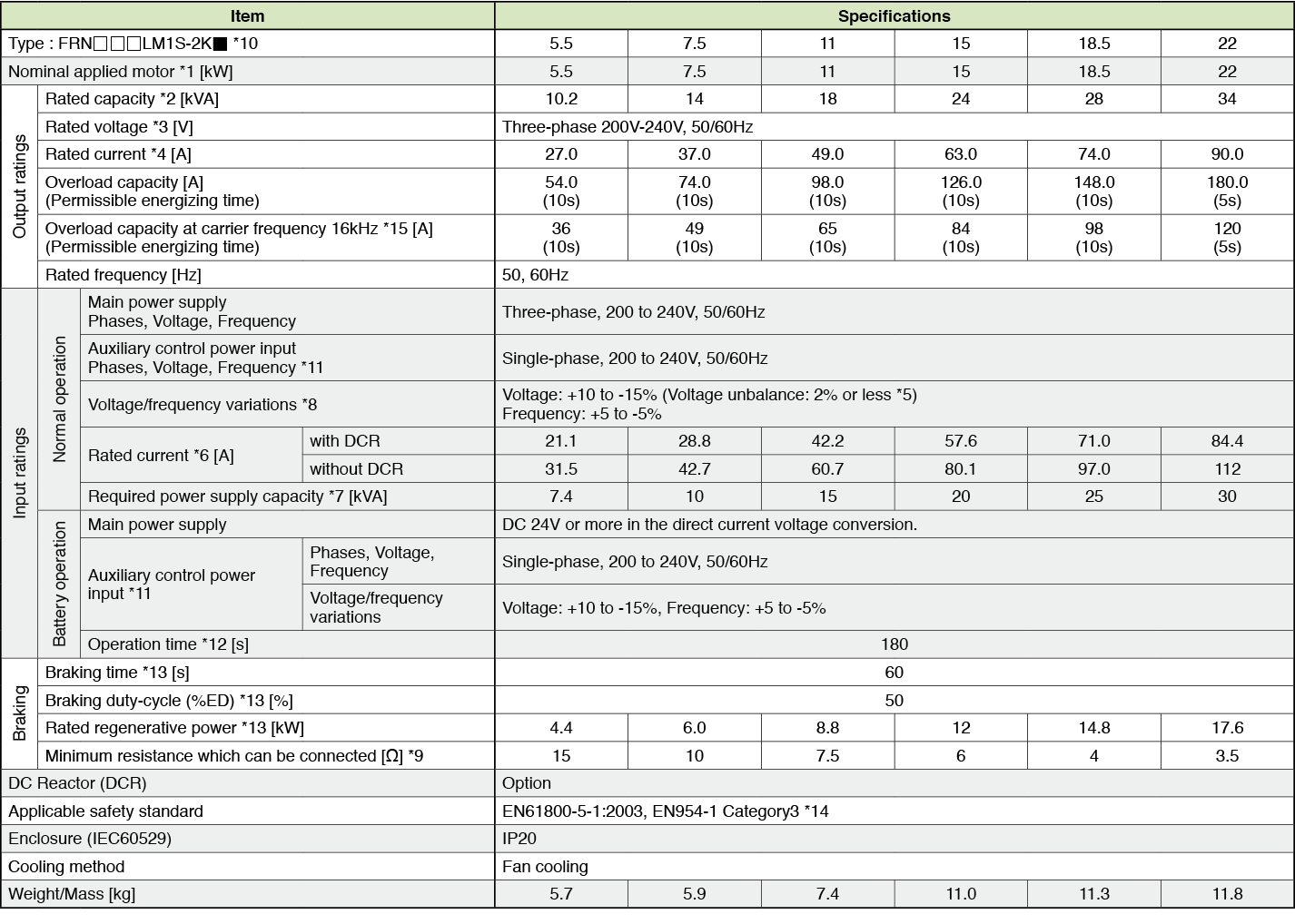*1)
Fuji’s 4-pole standard motor
*2)
Rated capacity is calculated by regarding the output rated voltage as 220V for three-phase 200V series.
*3)
Output voltage cannot exceed the power supply voltage.
*4)
It is a value in the condition of the career frequency 10kHz and the ambient temperature 45°C. Select the inverter capacity such that the square average current in cycle operation is 80% or less of the rated current of an inverter.
*5)
Voltage unbalance [%] = (Max. voltage [V] - Min. voltage [V])/ Three-phase average voltage [V] x 67 (IEC61800-3)
*6)
The power supply capacity is 500kVA (ten times the inverter capacity when the inverter capacity exceeds 50kVA), and the calculation value when connecting with the power supply of %X=5%.
*7)
Obtained when a DC Reactor is used.
*8)
An acceptable variation of the main power supply and the control power supply assistance input.
*9)
The admissible error of minimum resistance is ±5%.
*10)
 Type of inverter Description FRN□□□LM1S-2K CAN version FRN□□□LM1S-2KA DCP version
*11)
The same AC power as the main power supply input is connected for the backup of the control circuit power source.
*12)
It is a value in the condition of the career frequency 10kHz and the ambient temperature 45°C. Use the inverter such that the square average current in battery operation is 80% or less of the rated current of an inverter.
*13)
Braking time and duty cycle (%ED) are defined by cycle operation at the rated regenerative power as shown in the figure below.
*14)
The inverter that last 2 digits of a software version are from 50 to 99 corresponds to this standard.
*15)
When output exceeds this overload capacity at carrier frequency 16kHz, carrier frequency is reduced automatically. The reduced carrier frequency is maintained until an inverter stops.

### Three-phase 400V series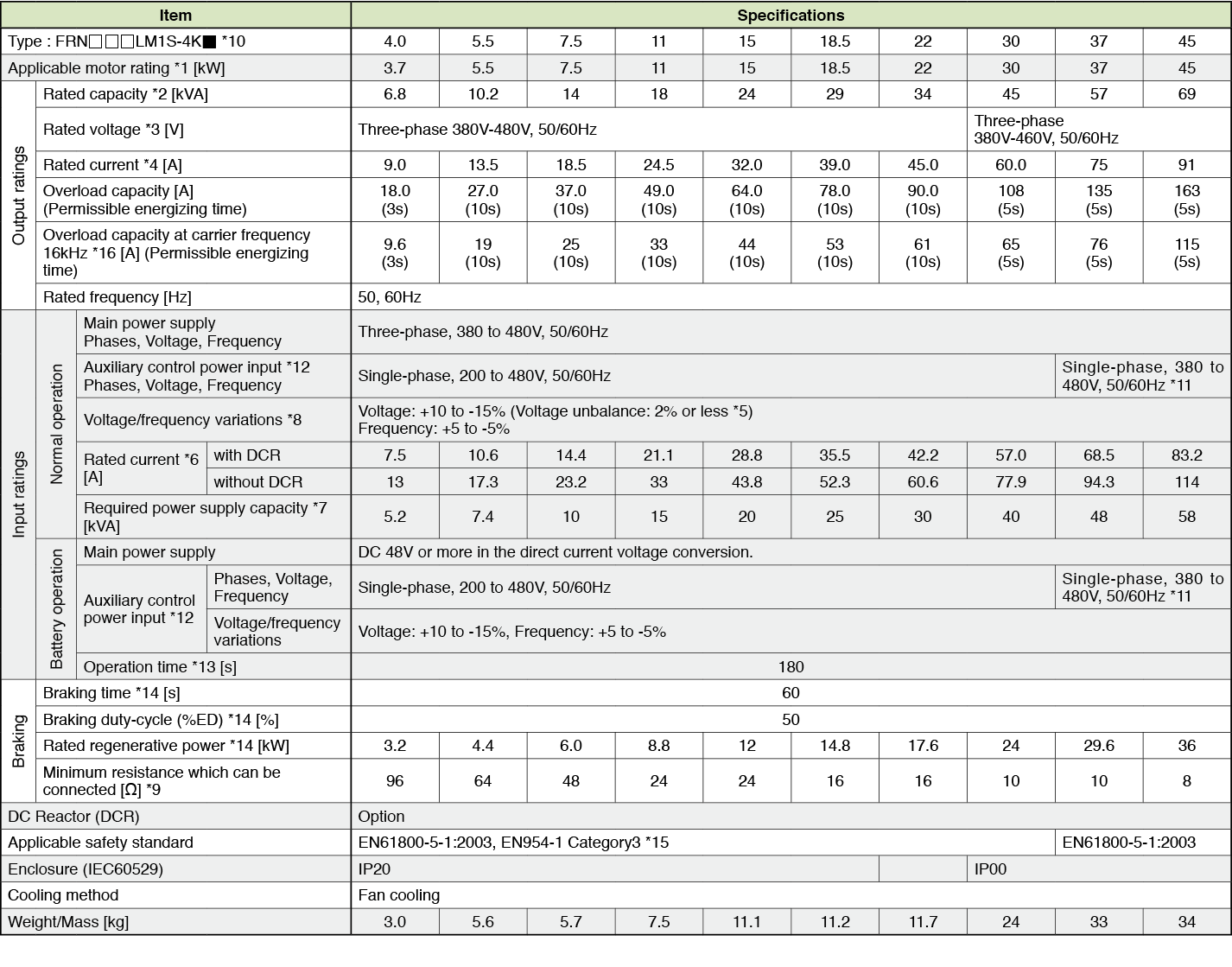*1)
Fuji’s 4-pole standard motor
*2)
Rated capacity is calculated by regarding the output rated voltage as 440V for three-phase 400V series.
*3)
Output voltage cannot exceed the power supply voltage.
*4)
It is a value in the condition of the career frequency 10kHz and the ambient temperature 45°C. Select the inverter capacity such that the square average current in cycle operation is 80% or less of the rated current of an inverter.
*5)
Voltage unbalance [%] = (Max. voltage [V] - Min. voltage [V])/ Three-phase average voltage [V] x 67 (IEC61800-3)
*6)
The power supply capacity is 500kVA (ten times the inverter capacity when the inverter capacity exceeds 50kVA), and the calculation value when connecting with the power supply of %X=5%.
*7)
Obtained when a DC Reactor is used.
*8)
An acceptable variation of the main power supply and the control power supply assistance input.
*9)
The admissible error of minimum resistance is ±5%.
*10)
 Type of inverter Description FRN□□□LM1S-4K CAN version FRN□□□LM1S-4KA DCP version
*11)
It is necessary to change the power-supply voltage change connector on the power supply printed wiring board depend on the power-supply voltage.
*12)
30kW or less
The same AC power as the main power supply input is connected for the backup of the control circuit power source.
37kW or more
The same AC power as the main power supply input is connected for the control circuit, the fan, and the contactor.
The inverter doesn’t operate if the power supply is not input to the auxiliary control power input. Please supply power.
*13)
It is a value in the condition of the career frequency 10kHz and the ambient temperature 45°C. Use the inverter such that the square average current in battery operation is 80% or less of the rated current of an inverter.
*14)
Braking time and duty cycle (%ED) are defined by cycle operation at the rated regenerative power as shown in the figure below.
*15)
The inverter that last 2 digits of a software version are from 50 to 99 corresponds to this standard.
*16)
When output exceeds this overload capacity at carrier frequency 16kHz, carrier frequency is reduced automatically. The reduced carrier frequency is maintained until an inverter stops.

### Single-phase 200V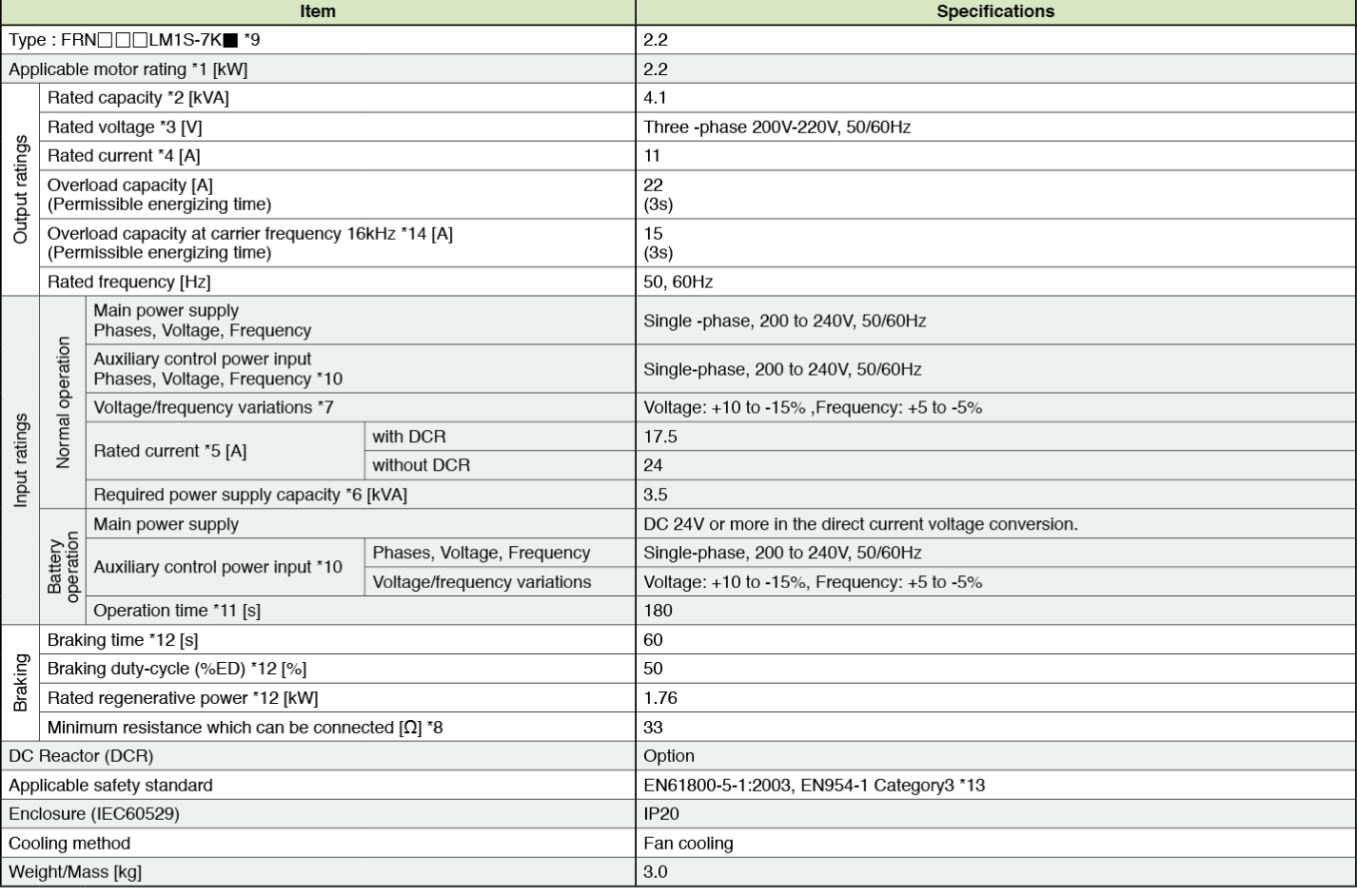*1)
Fuji’s 4-pole standard motor
*2)
Rated capacity is calculated by regarding the output rated voltage as 220V.
*3)
Output voltage cannot exceed the power supply voltage.
*4)
It is a value in the condition of the career frequency 10kHz and the ambient temperature 45°C. Select the inverter capacity such that the square average current in cycle operation is 80% or less of the rated current of an inverter.
*5)
The power supply capacity is 500kVA (ten times the inverter capacity when the inverter capacity exceeds 50kVA), and the calculation value when connecting with the power supply of %X=5%.
*6)
Obtained when a DC Reactor is used.
*7)
An acceptable variation of the main power supply and the control power supply assistance input.
*8)
The admissible error of minimum resistance is ±5%.
*9)
 Type of inverter Description FRN□□□LM1S-7K CAN version FRN□□□LM1S-7KA DCP version
*10)
The same AC power as the main power supply input is connected for the backup of the control circuit power source.
*11)
It is a value in the condition of the career frequency 10kHz and the ambient temperature 45°C. Use the inverter such that the square average current in battery operation is 80% or less of the rated current of an inverter.
*12)
Braking time and duty cycle (%ED) are defined by cycle operation at the rated regenerative power as shown in the figure below.*13)
The inverter that last 2 digits of a software version are from 50 to 99 corresponds to this standard.
*14)
When output exceeds this overload capacity at carrier frequency 16kHz, carrier frequency is reduced automatically. The reduced carrier frequency is maintained until an inverter stops.